Homework Help Question & Answers

# 3. Person A and B are both lawyers choosing between two career options: associate at a...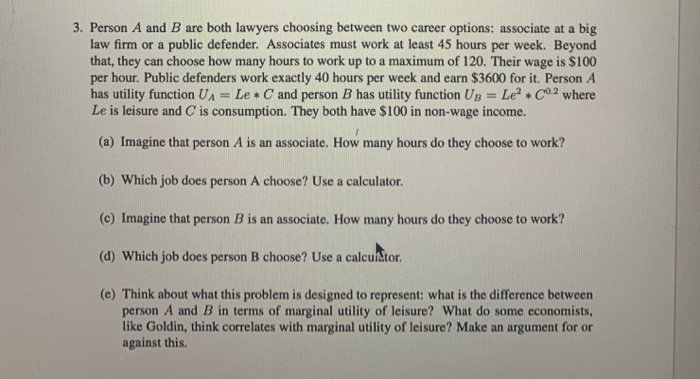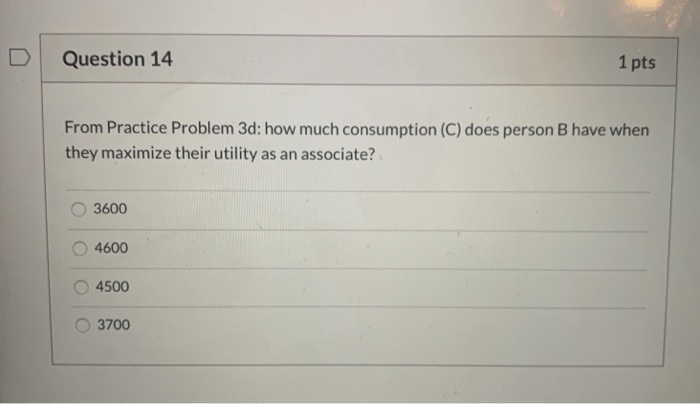3. Person A and B are both lawyers choosing between two career options: associate at a big law firm or a public defender. Associates must work at least 45 hours per week. Beyond that, they can choose how many hours to work up to a maximum of 120. Their wage is \$100 per hour. Public defenders work exactly 40 hours per week and earn \$3600 for it. Person A has utility function UA = Le* C and person B has utility function Up = Leº C0.2 where Le is leisure and C is consumption. They both have \$100 in non-wage income. (a) Imagine that person A is an associate. How many hours do they choose to work? (b) Which job does person A choose? Use a calculator. (©) Imagine that person B is an associate. How many hours do they choose to work? (d) Which job does person B choose? Use a calcuditor. (@) Think about what this problem is designed to represent: what is the difference between person A and B in terms of marginal utility of leisure? What do some economists. like Goldin, think correlates with marginal utility of leisure? Make an argument for or against this.
Question 14 1 pts From Practice Problem 3d: how much consumption (C) does person B have when they maximize their utility as an associate? 3600 4600 4500 3700

#### Homework Answers

Answer #1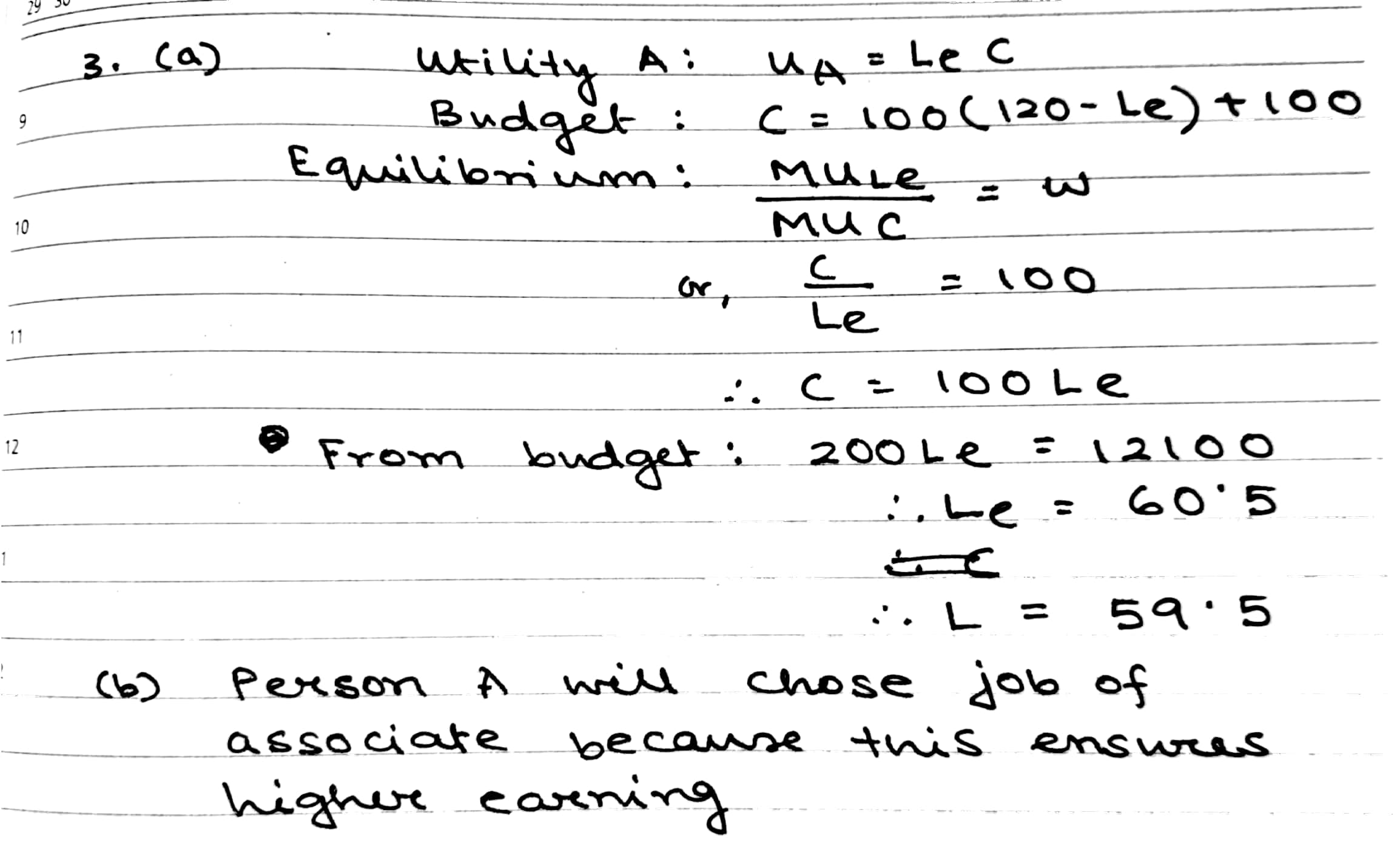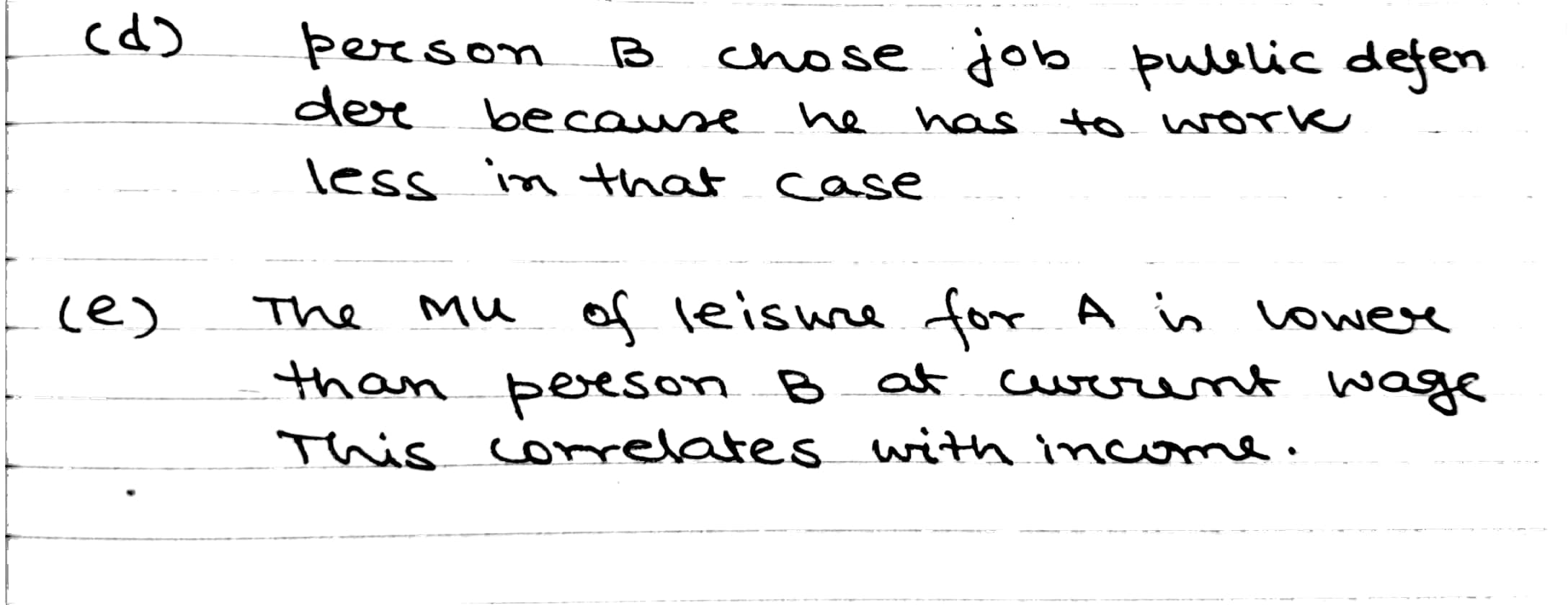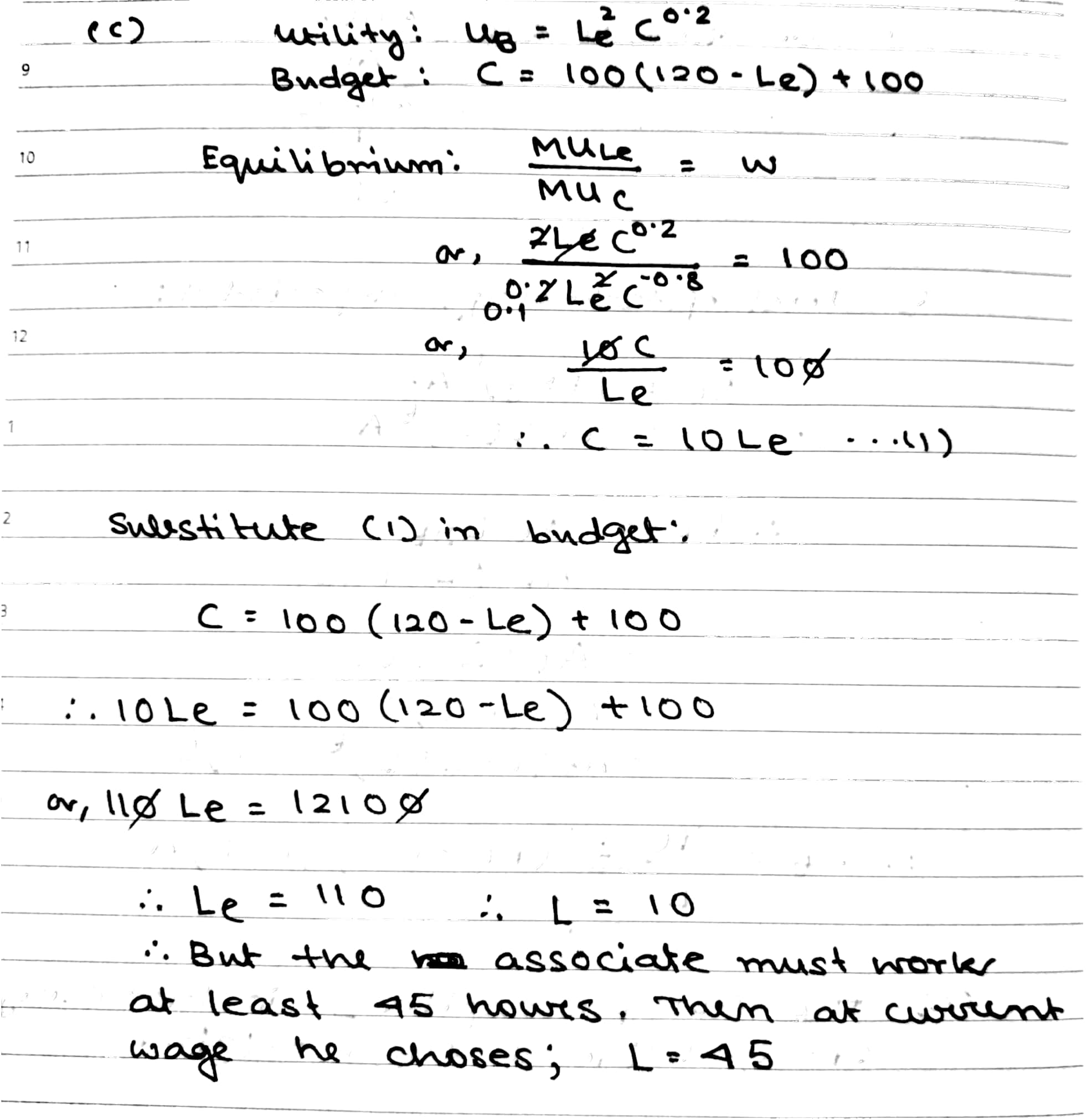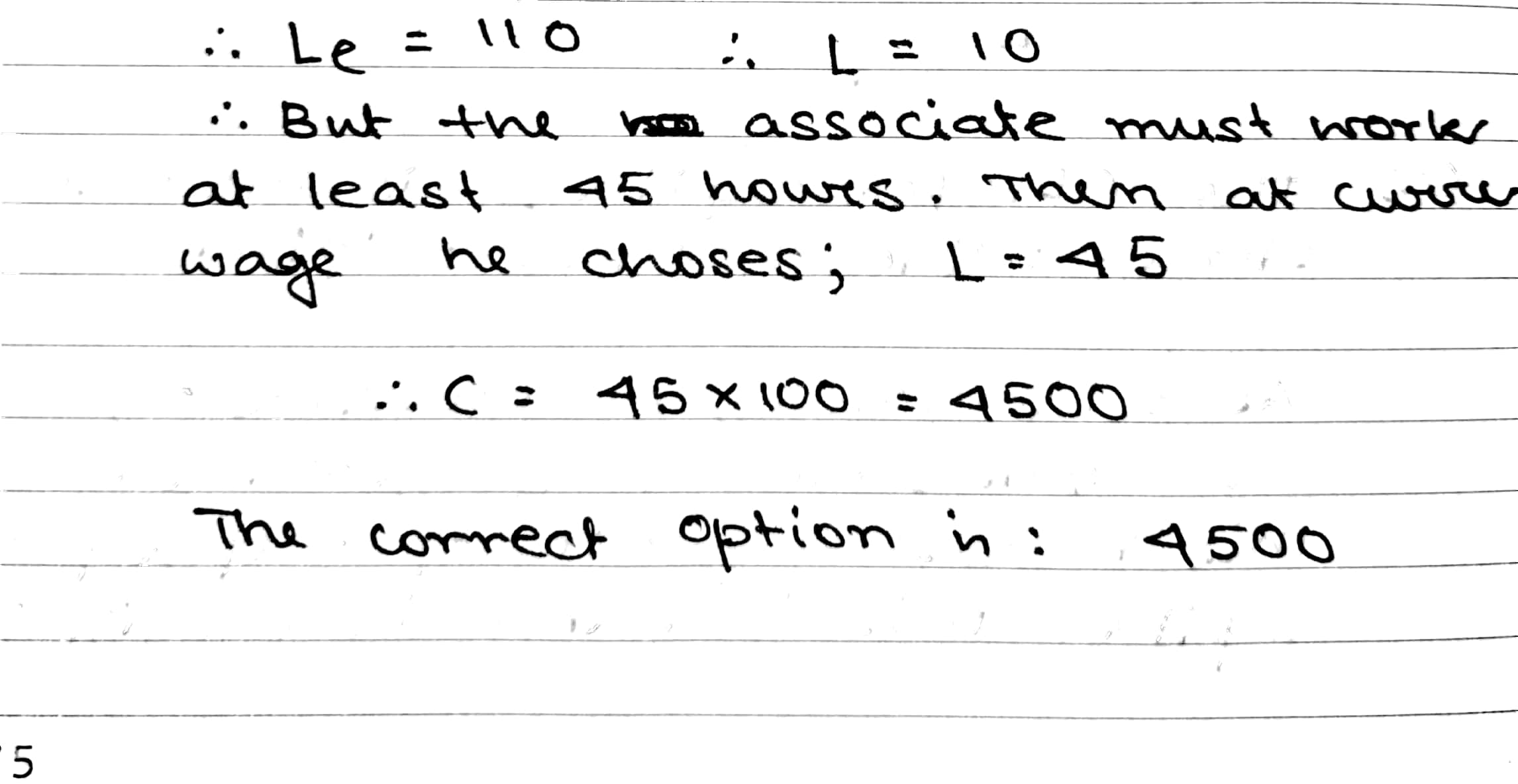Know the answer?
Your Answer:

#### Post as a guest

Your Name:

What's your source?

#### Earn Coin

Coins can be redeemed for fabulous gifts.

Not the answer you're looking for? Ask your own homework help question. Our experts will answer your question WITHIN MINUTES for Free.
Similar Homework Help Questions
• ### A person chooses between leisure and consumption. All of their consumption comes from current income. The...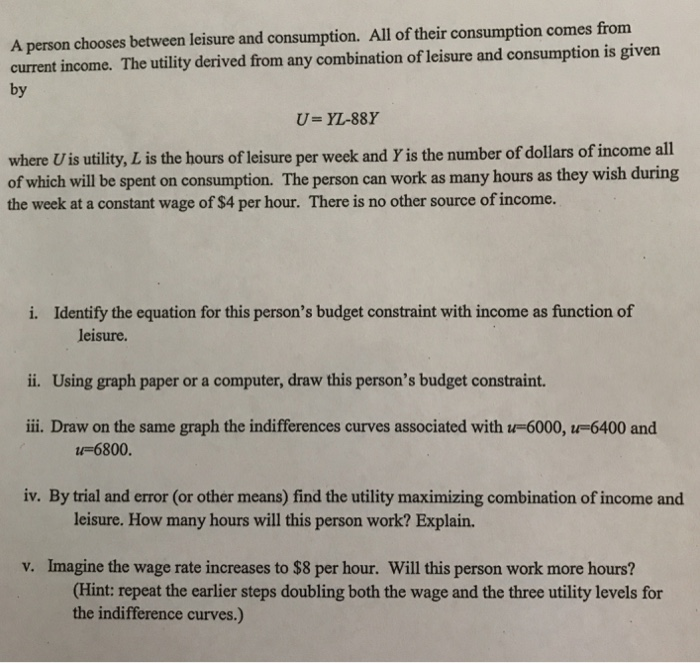A person chooses between leisure and consumption. All of their consumption comes from current income. The utility derived from any combination of leisure and consumption is given by U- YL-88Y where U is utility, L is the hours of leisure per week and Yis the number of dollars of income all of which will be spent on consumption. The person can work as many hours as they wish during the week at a constant wage of \$4 per hour. There...

• ### 2. Cindy gains utility from consumption C and leisure L. The most leisure she can consume...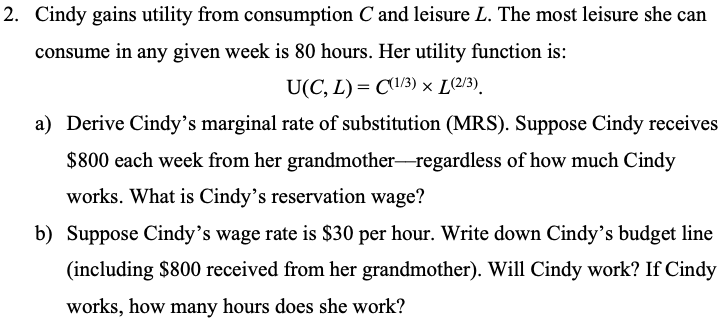2. Cindy gains utility from consumption C and leisure L. The most leisure she can consume in any given week is 80 hours. Her utility function is: U(CL)= (1/3) x L (2/3). a) Derive Cindy's marginal rate of substitution (MRS). Suppose Cindy receives \$800 each week from her grandmother-regardless of how much Cindy works. What is Cindy's reservation wage? b) Suppose Cindy's wage rate is \$30 per hour. Write down Cindy's budget line (including \$800 received from her grandmother). Will...

• ### Kirpa is trying to decide how many hours to work each week. Her utility is given by the following function: U(C,H) = C2 H3 , where C represents weekly consumption and H represents weekly leisure hours...

Kirpa is trying to decide how many hours to work each week. Her utility is given by the following function: U(C,H) = C2 H3 , where C represents weekly consumption and H represents weekly leisure hours. Her marginal utility with respect to consumption is MUc = 2CH3 , and her marginal utility with respect to leisure is MUH = 3C2 H2 . A) Find Kirpa's optimal H, L and C when w=\$7.50 and a = \$185. B) Suppose w increases...

• ### (6) Geo's utility function is described as LeY, where Le is hours of leisure per day,...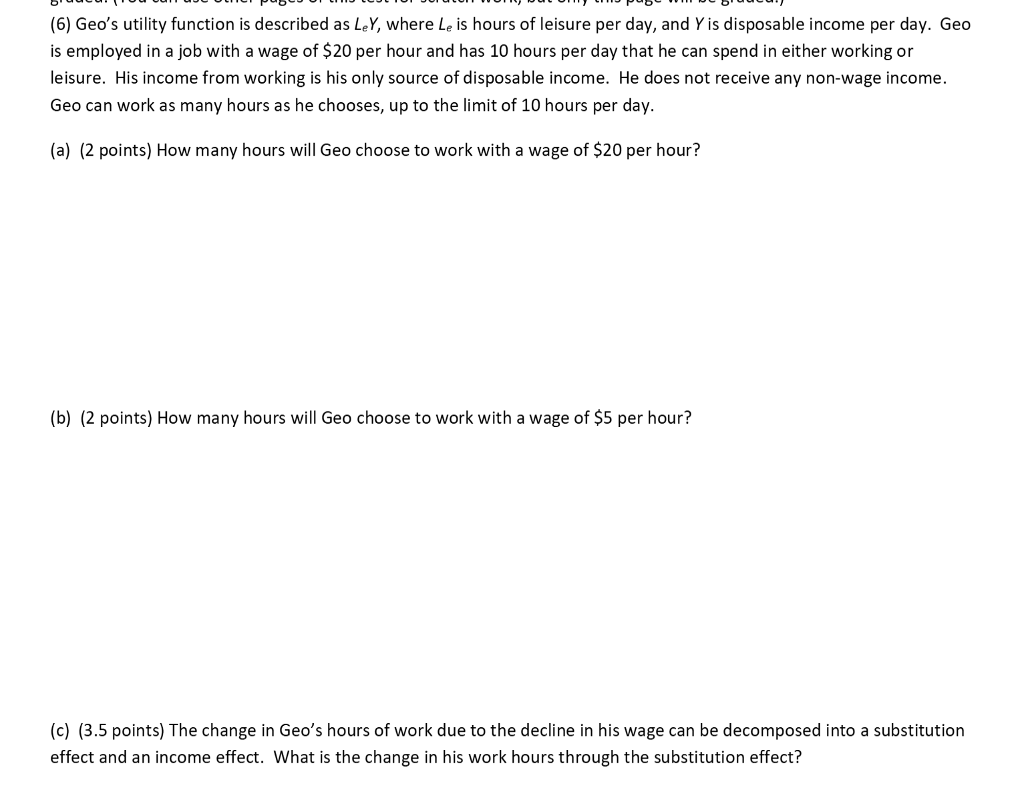(6) Geo's utility function is described as LeY, where Le is hours of leisure per day, and Y is disposable income per day. Geo is employed in a job with a wage of \$20 per hour and has 10 hours per day that he can spend in either working or leisure. His income from working is his only source of disposable income. He does not receive any non-wage income Geo can work as many hours as he chooses, up to...

• ### Jack gives each of his sister \$600 in non-labor income per week. Each sister has 100...

Jack gives each of his sister \$600 in non-labor income per week. Each sister has 100 hours per week to spend on labor or leisure, and each can earn a wage of \$30 per hour. part a. (4 points) Suppose Allison weekly utility function can be written as U=CL2, which gives her a marginal rate of substitution (MUL/MUC) equal to 2C/L. where C is the amount of consumption (in \$) and L is the hours of leisure she gets in...

• ### Jack gives each of his sister \$600 in non-labor income per week. Each sister has 100...

Jack gives each of his sister \$600 in non-labor income per week. Each sister has 100 hours per week to spend on labor or leisure, and each can earn a wage of \$30 per hour. part a. (4 points) Allison utility is more accurately represented by the function U=CL2, which gives her a marginal rate of substitution (MUL/MUC) equal to 2C/L. where C is the amount of consumption (in \$) and L is the hours of leisure she gets in...

• ### Problem 2 (30 points): Kirpa is trying to decide how many bours to work each week. Her utlity is ...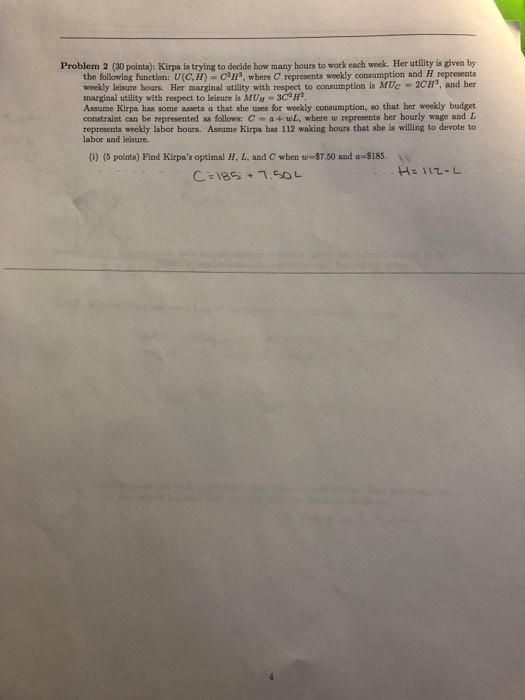Please be clear. Thank you! Problem 2 (30 points): Kirpa is trying to decide how many bours to work each week. Her utlity is given by the following function: U(C,H)CH3, where C represents weekly consumption and H represents weekly leisure bours. Her marginal utility with respect to consumption is MUc -2cH, and her marginal utility with respect to leisure is MUH 3C3H Assume Kirpa has some assets a that she uses for weekly consumption, so that her weekly budget constralnt...

• ### Leisure-labour choice 1. Mr. Cog works in a machine factory. He can work as many hours per day as he wishes at a wa...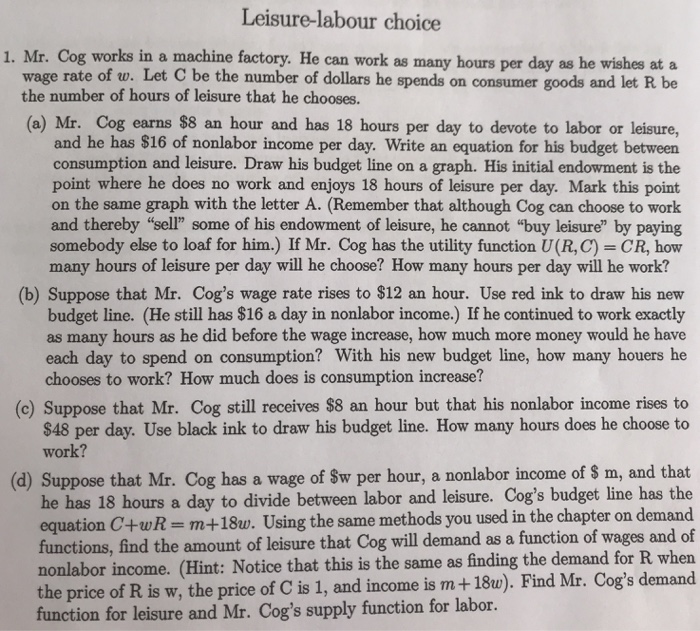Leisure-labour choice 1. Mr. Cog works in a machine factory. He can work as many hours per day as he wishes at a wage rate of w. Let C be the number of dollars he spends on consumer goods and let R be the number of hours of leisure that he chooses. (a) Mr. Cog earns \$8 an hour and has 18 hours per day to devote to labor or leisure, and he has \$16 of nonlabor income per day....

• ### 3. What happens to the reservation wage if nonlabor income increases, and why? You should include...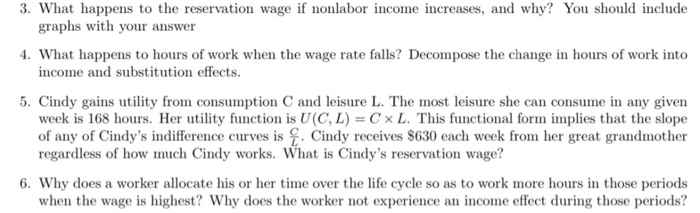3. What happens to the reservation wage if nonlabor income increases, and why? You should include graphs with your answer 4. What happens to hours of work when the wage rate falls? Decompose the change in hours of work into income and substitution effects. 5. Cindy gains utility from consumption C and leisure L. The most leisure she can consume in any given week is 168 hours. Her utility function is UCL) = CXL. This functional form implies that the...

• ### 3. What happens to the reservation wage if nonlabor income increases, and why? You should include...3. What happens to the reservation wage if nonlabor income increases, and why? You should include graphs with your answer 4. What happens to hours of work when the wage rate falls? Decompose the change in hours of work into income and substitution effects. 5. Cindy gains utility from consumption C and leisure L. The most leisure she can consume in any given week is 168 hours. Her utility function is UCL) = CXL. This functional form implies that the...

Free Homework App

Scan Your Homework
to Get Instant Free Answers
Need Online Homework Help?

Get Answers For Free
Most questions answered within 3 hours.